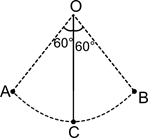A small sphere is suspended by a string of length l. The sphere is making oscillatory motion between extreme positions A and B then

# A small sphere is suspended by a string of length l. The sphere is making oscillatory motion between extreme positions A and B then1. A

Rate of work done by the gravitational force is zero at A, B and C.

2. B

work done by the tension in the string is always zero

3. C

both 1 and 2 are wrong

4. D

both 1 and 2 are right

Register to Get Free Mock Test and Study Material

+91

Verify OTP Code (required)

### Solution:

At the extreme positions A and B, velocity is zero. Tension induced in the string is always perpendicular to the direction of motion. Since power delivered by tension is $\stackrel{\to }{\mathrm{T}}.\stackrel{\to }{\mathrm{V}}$, it is always zero.

Power delivered by gravitational force is ${\stackrel{\to }{\mathrm{F}}}_{\mathrm{g}}.\stackrel{\to }{\mathrm{V}}$. At position C, $\stackrel{\to }{{\mathrm{F}}_{\mathrm{g}}}$ and $\stackrel{\to }{\mathrm{V}}$ are mutually perpendicular and at the extreme positions $\stackrel{\to }{\mathrm{V}}$ is zero. So option (1) is also right. Therefore, (4) is the correct option.Register to Get Free Mock Test and Study Material

+91

Verify OTP Code (required)